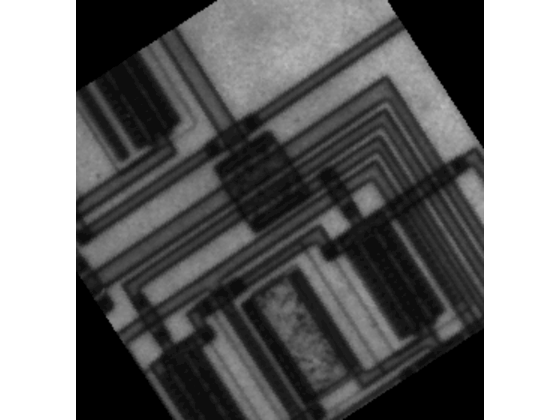Documentation

# houghlines

Extract line segments based on Hough transform

## Syntax

lines = houghlines(BW,theta,rho,peaks)
lines = houghlines(___,Name,Value,...)

## Description

example

lines = houghlines(BW,theta,rho,peaks) extracts line segments in the image BW associated with particular bins in a Hough transform. theta and rho are vectors returned by function hough. peaks is a matrix returned by the houghpeaks function that contains the row and column coordinates of the Hough transform bins to use in searching for line segments. The return value lines is a structure array whose length equals the number of merged line segments found.

example

lines = houghlines(___,Name,Value,...) extracts line segments in the image BW, where named parameters affect the operation.

## Examples

collapse all

Read image into workspace.

I = imread('circuit.tif');

Rotate the image.

rotI = imrotate(I,33,'crop');

Create a binary image.

BW = edge(rotI,'canny');

Create the Hough transform using the binary image.

[H,T,R] = hough(BW); imshow(H,[],'XData',T,'YData',R,... 'InitialMagnification','fit'); xlabel('\theta'), ylabel('\rho'); axis on, axis normal, hold on;Find peaks in the Hough transform of the image.

P = houghpeaks(H,5,'threshold',ceil(0.3*max(H(:)))); x = T(P(:,2)); y = R(P(:,1)); plot(x,y,'s','color','white');Find lines and plot them.

lines = houghlines(BW,T,R,P,'FillGap',5,'MinLength',7); figure, imshow(rotI), hold on max_len = 0; for k = 1:length(lines) xy = [lines(k).point1; lines(k).point2]; plot(xy(:,1),xy(:,2),'LineWidth',2,'Color','green'); % Plot beginnings and ends of lines plot(xy(1,1),xy(1,2),'x','LineWidth',2,'Color','yellow'); plot(xy(2,1),xy(2,2),'x','LineWidth',2,'Color','red'); % Determine the endpoints of the longest line segment len = norm(lines(k).point1 - lines(k).point2); if ( len > max_len) max_len = len; xy_long = xy; end endHighlight the longest line segment by coloring it cyan.

plot(xy_long(:,1),xy_long(:,2),'LineWidth',2,'Color','cyan');## Input Arguments

collapse all

Binary image, specified as a real, 2-D, nonsparse logical or numeric array.

Data Types: single | double | int8 | int16 | int32 | int64 | uint8 | uint16 | uint32 | uint64 | logical

Line rotation angle in radians, specified as a real, 2-D, nonsparse logical or numeric array.

Data Types: double

Distance from the coordinate origin, specified as a real, 2-D, nonsparse logical or numeric array. The coordinate origin is the top-left corner of the image (0,0).

Data Types: double

Row and column coordinates of Hough transform bins, specified as a real, nonsparse numeric array.

Data Types: double

### Name-Value Pair Arguments

Specify optional comma-separated pairs of Name,Value arguments. Name is the argument name and Value is the corresponding value. Name must appear inside quotes. You can specify several name and value pair arguments in any order as Name1,Value1,...,NameN,ValueN.

Example: lines = houghlines(BW,T,R,P,'FillGap',5,'MinLength',7);

Distance between two line segments associated with the same Hough transform bin, specified as a positive real scalar. When the distance between the line segments is less than the value specified, the houghlines function merges the line segments into a single line segment.

Data Types: double

Minimum line length, specified as a positive real scalar. houghlines discards lines that are shorter than the value specified.

Data Types: double

## Output Arguments

collapse all

Lines found, returned as a structure array whose length equals the number of merged line segments found. Each element of the structure array has these fields:

Field

Description

point1

Two element vector [X Y] specifying the coordinates of the end-point of the line segment

point2

Two element vector [X Y] specifying the coordinates of the end-point of the line segment

theta

Angle in degrees of the Hough transform bin

rho

rho axis position of the Hough transform bin